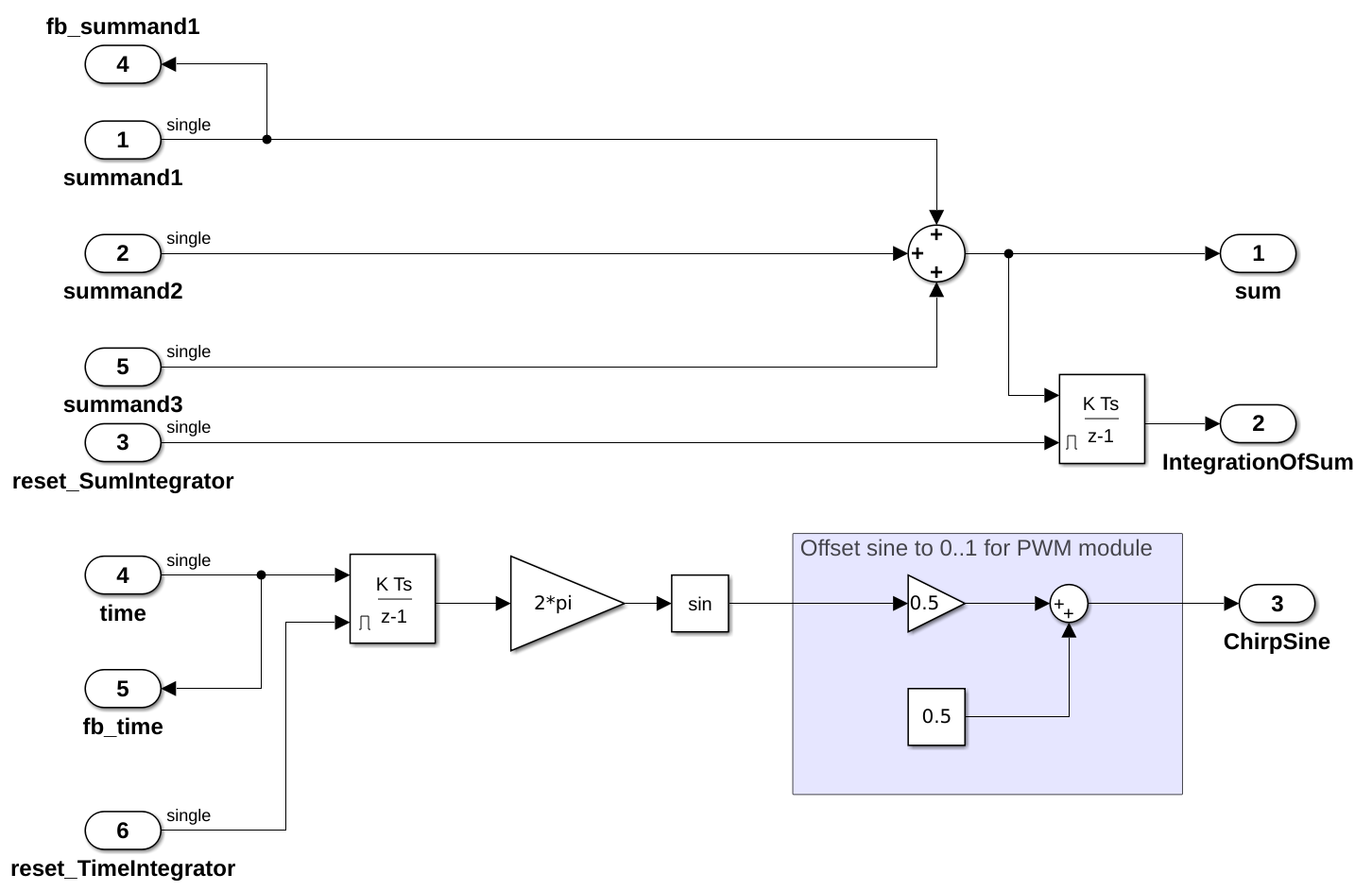# How to create framework functions from Embedded Coder

The functions of the UltraZohm Software Framework follow the principles outlined in Software Development Guidelines. Encapsulation and data hiding are important characteristics for these software modules. C-Code that is generated by using the Embedded-Coder (C-Code) is not directly compatible with the software guidelines and require a wrapper module to be included in the software framework. The guiding principle is that the interface has to be consistent across modules, since it is not relevant whether the code is handwritten or auto-generated.

The following guide builds on the example model of Embedded-Coder (C-Code) and highlight how to create an interface that is consistent with the Software Development Guidelines (multiple-instance module).

## Functional description of the moduleFig. 36 Screenshot of the subsystem that is used for code generation in the example of Embedded-Coder (C-Code)

Fig. 36 shows the subsystem that is code generated in Embedded-Coder (C-Code). The following guide only implements an interface for the sum functionality of the module. Thus, the code ought to calculate:

$\begin{split}y &= a + b + c \\ y_2 &=\int y\end{split}$

The Simulink model uses forward Euler integration (see Matlab documentation discrete integrator). Note that the integration time $$T_s=\frac{1}{10000}=0.0001$$ is hard-coded in the module, due to the model settings in Embedded-Coder (C-Code). The chirp function is not used in this guide, but can be implemented with the same principles.

## Create framework function

Note

This guide uses the already generated files of the example in Embedded-Coder (C-Code). For custom modules, this step has to be adjusted to match the path of the specific embedded coder settings!

1. Open ultrazohm_sw with VSCode and remote container (see VS Code Remote Container)

2. Create a new module uz_sum using Ceedling

cd vitis/software/Baremetal
ceedling module:create[uz/uz_sum/uz_sum]

1. Ceedling creates the header, source, and test files

2. Copy the generated files to uz_sum, e.g., by using the following command:

cp -R src/Codegen/uz_codegen0_ert_rtw/ src/uz/uz_sum/


Warning

Re-using this module with the same name breaks the software. In the finished code of this how to guide, everything in uz_sum folder is protected by #ifdef TEST such that it can only be used in the ceedling unit tests.

1. Create the allocation scheme using the allocation snippet (see Static memory allocation)

#include "../uz_global_configuration.h"
#if UZ_SUM_MAX_INSTANCES > 0U

#include <stdbool.h>
#include "../../uz_HAL.h"
#include "uz_sum.h"

struct uz_sum_t {
};

static uint32_t instance_counter = 0U;
static uz_sum_t instances[UZ_SUM_MAX_INSTANCES] = { 0 };

static uz_sum_t* uz_sum_allocation(void);

static uz_sum_t* uz_sum_allocation(void){
uz_assert(instance_counter < UZ_SUM_MAX_INSTANCES);
uz_sum_t* self = &instances[instance_counter];
instance_counter++;
return (self);
}

uz_sum_t* uz_sum_init(void) {
uz_sum_t* self = uz_sum_allocation();
return (self);
}

#endif

1. Call the module uz_sum

2. Add UZ_SUM_MAX_INSTANCES to uz_global_configuration.h and set it to 5

3. Add the typedef for the uz_sum_t to uz_sum.h as well as the function declaration for the init function:

typedef struct uz_sum_t uz_sum_t;
uz_sum_t* uz_sum_init(void);

1. In test_uz_sum.c, change the existing test to:

void test_uz_sum_NeedToImplement(void)
{
uz_sum_init();
}

1. Run the tests, they compile but test_uz_sum.c does not perform any real tests

2. Create the interface for stepping the model once (one integration / time step) with the given summand in uz_sum.h:

void uz_sum_step(uz_sum_t* self, float a, float b, float c);

1. Add an interface for reading the results from the module in uz_sum.h

float uz_sum_get_sum(uz_sum_t* self);
float uz_sum_get_integral_over_sum(uz_sum_t* self);

1. Write empty functions for the defined interface in uz_sum.c

void uz_sum_step(uz_sum_t* self, float a, float b, float c){

}

float uz_sum_get_sum(uz_sum_t* self){

}

float uz_sum_get_integral_over_sum(uz_sum_t* self){

}

1. Write a test that checks for the summation of three values:

void test_uz_sum_add_numbers(void)
{
uz_sum_t* test_instance=uz_sum_init();
float a=1.1f;
float b=2.2f;
float c=3.3f;
float expected_result=6.6f;

uz_sum_step(test_instance,a,b,c);
float result=uz_sum_get_sum(test_instance);
TEST_ASSERT_EQUAL_FLOAT(expected_result, result);

}

1. Run the tests. They will compile, but fail.

2. Add the include for the generated code as well as private data to uz_sum.c (note: this has to be in the .c file!)

#include "uz_codegen0_ert_rtw/uz_codegen0.h"

struct uz_sum_t {
ExtY output;
ExtU input;
DW rtDW;                        /* Observable states */
RT_MODEL modelData;
RT_MODEL *PtrToModelData;
};

1. Implement the initialization of the code-generated software in uz_sum_init.c

uz_sum_t* uz_sum_init(void) {
uz_sum_t* self = uz_sum_allocation();
self->PtrToModelData=&self->modelData;
self->PtrToModelData->dwork=&self->rtDW;
self->PtrToModelData->inputs=&self->input;
self->PtrToModelData->outputs=&self->output;
return (self);
}

1. Note that uz_sum_init is just wiring of private variables of the module to meet the interface of the generated code and to be able to pass all data of the model to the step function by a single pointer.

2. Add #include "uz_codegen0_ert_rtw/uz_codegen0.h" to test_uz_sum.c to enable calling the generated code in the tests

3. Implement the function uz_sum_step in uz_sum.c:

void uz_sum_step(uz_sum_t* self, float a, float b, float c){
self->input.summand1=a;
self->input.summand2=b;
self->input.summand3=c;
uz_codegen0_step(self->PtrToModelData);
}

1. Run the tests, they still fail.

2. Implement uz_sum_get_sum in uz_sum.c

float uz_sum_get_sum(uz_sum_t* self){
return self->output.sum;
}

1. Run tests, they pass.

2. Write a test for the integration

void test_uz_sum_integrate(void)
{
uz_sum_t* test_instance=uz_sum_init();
float a=1.1f;
float b=2.2f;
float c=3.3f;
float expected_result=0.00198f;

// Call step four times with sum=6.6, integration time Ts is 1/10000
// First call: y(0)=0
// Second call: y(1)= 1/10000*6.6=0.00066
// Third call: y(2)=0.00066+0.00066=0.00132
// Last call: y(3)=0.00132+0.00066=0.00198
// Step four times - no loop to make it explicit
uz_sum_step(test_instance,a,b,c);
uz_sum_step(test_instance,a,b,c);
uz_sum_step(test_instance,a,b,c);
uz_sum_step(test_instance,a,b,c);
float result=uz_sum_get_integral_over_sum(test_instance);
TEST_ASSERT_EQUAL_FLOAT(expected_result,result);
}

1. Run tests, they fail.

2. Implement uz_sum_get_integral_over_sum

float uz_sum_get_integral_over_sum(uz_sum_t* self){
return self->output.IntegrationOfSum;
}

1. Run tests, they pass.

2. Implement tests and interface for the chirp functionality

3. Write documentation for the software module

Note

The source Simulink model that is used for generating the code should be supplied in the same folder as the generated code. Do not commit anything expect .slx, .c, and .h files after the code generation, i.e., no Simulink cache or other build artifacts!